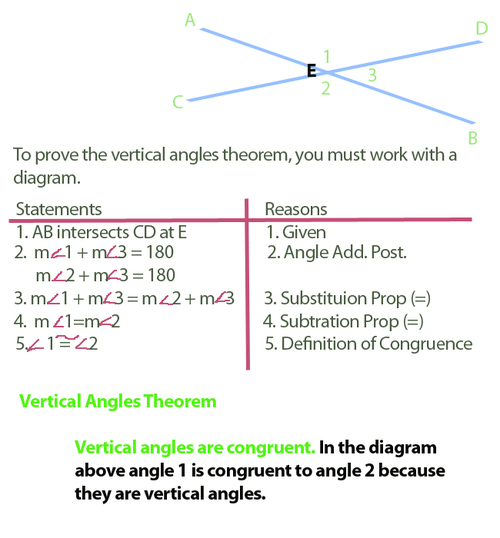Proofs
3 years ago
jpaluch
Save
Edit
Host a game
Live GameLive
Homework
Solo Practice
Practice20 QuestionsShow answers
• Question 1
30 seconds
Q. Rigid Motions preserve ...
size and shape
size and location
shape and location
shape and direction
• Question 2
60 secondsQ. What is the justification (reason)?
Reflexive Property
Symmetric Property
Transitive Property
Substitution
• Question 3
60 secondsQ. What is the justification (reason)?
reflexive property
definition of segment bisect
definition of a midpoint
substitution property
• Question 4
30 seconds
Q. Which of the following is NOT a triangle congruence criteria?
SAS
AAS
SSA
ASA
• Question 5
30 seconds
Q. What does CPCTC stand for?
Corresponding Parts of Congruent Triangles are Congruent
Congruent Parts of Congruent Triangles are Corresponding
Colorful Pieces of Corresponding Triangles are Congruent
Congruent Pieces of Congruent Triangles are Congruent
• Question 6
30 seconds
Q. A parallelogram is a rectangle when the diagonals ...
are perpendicular.
bisect each other.
are congruent.
are parallel.
• Question 7
30 seconds
Q. When using hypotenuse leg (HL) in a proof, you must first state ...
CPCTC.
there are right triangles.
that vertical angles are congruent.
the reflexive property.
• Question 8
30 secondsQ. What triangle congruence criteria is shown in the given diagram?
AAS
ASA
SSA
SAS
• Question 9
30 secondsQ. What triangle congruence criteria is shown in the given diagram?
SSS
SAS
ASA
AAS
• Question 10
30 secondsQ. What triangle congruence criteria is shown in the given diagram?
ASA
AAS
SSA
HL
• Question 11
30 seconds
Q. A triangle with two congruent sides and two congruent angles (base angles) is called ...
scalene
equilateral
isosceles
obtuse
• Question 12
30 seconds
Q. A centroid is formed by the point of concurrency of three __________ of a triangle.
altitudes
medians
perpendicular bisectors
angle bisectors
• Question 13
30 seconds
Q. A median of a triangle connects the ___________ of a side to the opposite vertex.
midpoint
right angle
end point
angle
• Question 14
30 seconds
Q. A centroid breaks the median of a triangle into a ________ ratio.
1:1
2:1
3:1
4:1
• Question 15
30 secondsQ. If point B is the centroid and BC = 12, what is the length of AB?
12
8
6
4
• Question 16
30 seconds
Q. By definition, a parallelogram ...
has opposite sides that are parallel.
has four sides.
is a slanted rectangle.
has four right angles.
• Question 17
30 secondsQ. What is the missing piece of information required to prove these triangles congruent?
QY ≅ QY
NY ≅ PY
∠N ≅ ∠P
QY is the perpendicular bisector
• Question 18
30 seconds
Q. Which quadrilateral has diagonals that are always perpendicular bisectors of each other?
trapezoid
parallelogram
rectangle
square
• Question 19
30 seconds
Q. The orthocenter is the point of concurrency for three ...
perpendicular bisectors
angle bisectors
medians
altitudes
• Question 20
30 seconds
Q. Point B (2, -3) is rotated 90° about the origin.  What are the coordinates of B'?